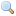# Module Random

`module Random: `sig` .. `end``
Pseudo-random number generators (PRNG).

###### Basic functions

`val init : `int -> unit``
Initialize the generator, using the argument as a seed. The same seed will always yield the same sequence of numbers.
`val full_init : `int array -> unit``
Same as `Random.init` but takes more data as seed.
`val self_init : `unit -> unit``
Initialize the generator with a random seed chosen in a system-dependent way. If `/dev/urandom` is available on the host machine, it is used to provide a highly random initial seed. Otherwise, a less random seed is computed from system parameters (current time, process IDs).
`val bits : `unit -> int``
Return 30 random bits in a nonnegative integer.
Before 3.12.0 used a different algorithm (affects all the following functions)
`val int : `int -> int``
`Random.int bound` returns a random integer between 0 (inclusive) and `bound` (exclusive). `bound` must be greater than 0 and less than 230.
`val int32 : `Int32.t -> Int32.t``
`Random.int32 bound` returns a random integer between 0 (inclusive) and `bound` (exclusive). `bound` must be greater than 0.
`val nativeint : `Nativeint.t -> Nativeint.t``
`Random.nativeint bound` returns a random integer between 0 (inclusive) and `bound` (exclusive). `bound` must be greater than 0.
`val int64 : `Int64.t -> Int64.t``
`Random.int64 bound` returns a random integer between 0 (inclusive) and `bound` (exclusive). `bound` must be greater than 0.
`val float : `float -> float``
`Random.float bound` returns a random floating-point number between 0 and `bound` (inclusive). If `bound` is negative, the result is negative or zero. If `bound` is 0, the result is 0.
`val bool : `unit -> bool``
`Random.bool ()` returns `true` or `false` with probability 0.5 each.

The functions from module `State` manipulate the current state of the random generator explicitly. This allows using one or several deterministic PRNGs, even in a multi-threaded program, without interference from other parts of the program.
`module State: `sig` .. `end``
`val get_state : `unit -> State.t``
`val set_state : `State.t -> unit``